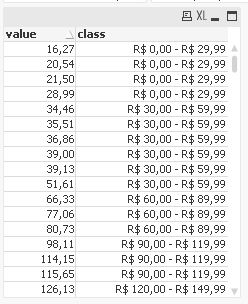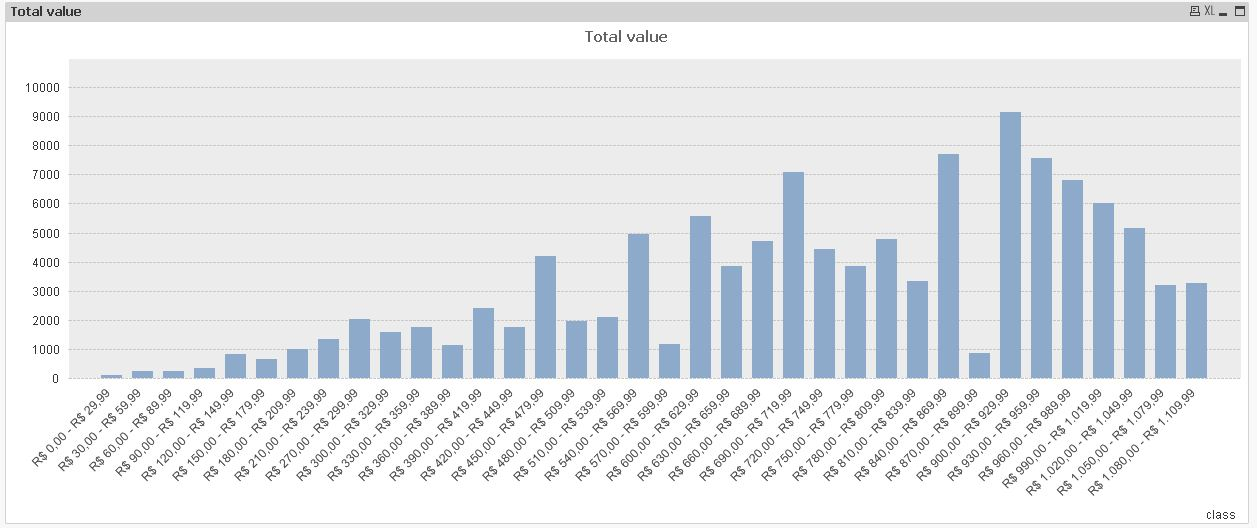# QlikView Scripting

Discussion Board for collaboration on QlikView Scripting.

Announcements

Contributor

## class editavel

Boa tarde

É possível tratar diferente uma informação que foi formata pela função CLASS

por exemplo, para trazer normalmente ele traz

0.00 a 30.00

mas eu quero trazer o valor a direita desta forma

0.00 a 29.99

quando edito e faço na formula a função - 0.01

ele apresenta desta forma

-0.01 a 29.99

1 Solution

Accepted SolutionsMVP

## Re: class editavel

Boa tarde carla,

one solution for this question could be:

Dual(Num(SubField(Class(value,30),' <= x < ',1),'R\$ #.##0,00')&' - '&Num(SubField(Class(value,30),' <= x < ',2)-0.01,'R\$ #.##0,00'),Class(value,30))Due to the creation of dual values, you even don't have to care about sorting expressions:```table1:
Dual(Num(SubField(Class(value,30),' <= x < ',1),'R\$ #.##0,00')&' - '&Num(SubField(Class(value,30),' <= x < ',2)-0.01,'R\$ #.##0,00'),Class(value,30)) as class;
LOAD Round(Rand()*1100,0.01) as value
AutoGenerate 200;
```

hope this helps

regards

MarcoMVP

## Re: class editavel

Boa tarde carla,

one solution for this question could be:

Dual(Num(SubField(Class(value,30),' <= x < ',1),'R\$ #.##0,00')&' - '&Num(SubField(Class(value,30),' <= x < ',2)-0.01,'R\$ #.##0,00'),Class(value,30))Due to the creation of dual values, you even don't have to care about sorting expressions:```table1: